Reach Us+44 7482 874137
Model-Based Quantification of Blood Flow Rate and Oxygen Consumption Rate of Biological Tissues Using Image-Guided Near Infrared Light Spectroscopy | OMICS International
Journal of Biosensors & Bioelectronics

Like us on:

All submissions of the EM system will be redirected to Online Manuscript Submission System. Authors are requested to submit articles directly to Online Manuscript Submission System of respective journal.

# Model-Based Quantification of Blood Flow Rate and Oxygen Consumption Rate of Biological Tissues Using Image-Guided Near Infrared Light Spectroscopy

Zhen Yuan*

Faculty of Health Sciences, University of Macau, Av. Padre Tomás Pereira, Taipa, Macau, China

*Corresponding Author:
Zhen Yuan
Assistant Professor of Health Sciences
University of Macau
Av. Padre Tomás Pereira
Taipa, Macau, China
E-mail: [email protected]

Received Date: March 19, 2013; Accepted Date: April 21, 2013; Published Date: April 23, 2013

Citation:Yuan Z (2013) Model-Based Quantification of Blood Flow Rate and Oxygen Consumption Rate of Biological Tissues Using Image-Guided Near Infrared Light Spectroscopy. J Biosens Bioelectron 4:135. doi: 10.4172/2155-6210.1000135

Copyright: © 2013 Yuan Z. This is an open-access article distributed under the terms of the Creative Commons Attribution License, which permits unrestricted use, distribution, and reproduction in any medium, provided the original author and source are credited.

Visit for more related articles at Journal of Biosensors & Bioelectronics

#### Abstract

Image-guided near infrared spectroscopy has been implemented to deduce the map of oxygen consumption rate and blood flow rate of biological tissues using a model-based method combined the noninvasive diffuse light measurement techniques. The developed method relies on optical imaging to recover the concentrations of oxygen and de-oxygen hemoglobin concentration of tissues, and the ordinary differential equations to recover the vascular parameters including oxygen consumption rate and blood flow rate. In particular, the mathematical model has been validated by both simulation and experimental tests.

#### Introduction

Analysis of the physiological responses of functional brain activation based on intrinsic optical signals has revealed new insights into the functional representations of areas such as the visual and somatosensory cortices [1,2]. In addition to hemoglobin change, cerebral blood flow rate (CBF) and oxygen consumption rate (OC) changes resulting from functional activation are also important components of the hemodynamic response. The nature of the coupling between neuronal activity and the associated hemodynamic response is now becoming an interesting subject in neuroscience [3,4]. Clearer understandings of the neuro-metabolic-vascular relationship will enable greater insight into the functioning of the normal brain and will also have significant impact on diagnosis and treatment of neurovascular diseases such as stroke, Alzheimer’s disease, and head injury and epilepsy . In order to achieve this goal, simultaneous monitoring of the spatiotemporal characteristics of OC, CBF, and the cerebral metabolic rate of oxygen is crucial.

Although many methods for assessment of cerebral OC and CBF have been explored including magnetic resonance imaging (MRI) , arterial spin labeling MRI , PET , Fick's lawbased optical systems , laser Doppler , diffuse correlation spectroscopy , and Doppler ultrasound , there remains a critical need for continuous, noninvasive instruments to measure CBF and OC in humans with intact skull. For example, the spatiotemporal resolution of PET is limited, and fMRI requires careful calibration of the scaling factor between the blood oxygen level dependent signal and the relative changes of de oxy hemoglobin concentration, as well as assumptions about the relationship between the changes in CBF and cerebral blood volume. Laser Doppler flow meter has limited penetration depth. Diffuse correlation spectroscopy has shown promising results, but it is still unclear whether it is practical enough to be used for continuous monitoring in humans. Fick's law-based systems are not entirely non invasive, since they require the injection of a chromophore, and therefore cannot be used for continuous monitoring. Laser speckle contrast imaging method can effectively recover the BF and OC parameters. However, due to the cost and complexity, laser speckle imaging setups may not be readily available to researchers .

On the other hand, due to numerous advantages of low cost, portability, and non-ionizing radiation, near-infrared (NIR) diffuse optical spectroscopy (DOS) and tomography (DOT) have offered a promising alternative for continuous bedside monitoring of patients . DOS and DOT have more than 30 year history of being used to access tissues total hemoglobin concentration, hemoglobin oxygen saturation, water and lipid concentration, tissue scattering, oxygen metabolism and blood flow [1,2,15-21]. NIR optical imaging modalities have shown a great potential to provide high spatiotemporal resolution and more quantitative imaging of hemodynamic responses . In particular, dynamic optical imaging has allowed the exploration of time-resolved changes in tissues properties. The dynamics measured with DOT in the functional neuro imaging are due to the dynamics in blood volume and oxygenation in the scalp and brain. And the hemodynamics is caused by CBF and OC change associate with heart beat, vasomotion and vascular response to neuronal activity . However, dynamic DOT imaging techniques provide only the change of total hemoglobin concentration (HbT) and oxygen saturation (SO2), which cannot give the change of CBF and OC due to the hemodynamic response to neuronal activation. As such, new mathematical models connecting changes in CBF and OC to observed changes in HbT and SO2 are required to guide more quantitative interpretation of neuronactivity. The model should be able to present indirect measurements of neuron-induced vascular parameters including blood flow (BF) and OC.

Developed mathematical models to reconstruct BF and OC of blood vessel and biological tissues

The technology now existing for obtaining quantitative information on how oxygen and blood are managed in the tissues is the principle of mass balance to the transport of oxygen in a blood vessel segment.  To model oxygen transport in a blood vessel by this principle, we consider a one-dimensional cylindrical vessel (blood vessel) with Ri and Ro as the inner and outer radii, respectively, surrounded by the biological tissues. In addition, we assume all the oxygen (O2) diffusing out the segment is consumed in a tissue region .

Mass balance in each segment for intravascular flux

The law of mass conservation stipulates that the amount of O2 lost from a vascular segment must be equal to the diffuse O2 flux to the tissues, determined by the perivascular oxygen gradients. For a steady case, we have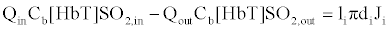(1)

in which Qin (mL.s-1) is the volumetric BF into the ith segment, Qout the volumetric BF out the segment, di is the diameter of the ith segment, li the length of the ith segment, HbT the total hemoglobin concentration in the blood (moles), SO2,in the hemoglobin oxygen saturation flowing in the segment, SO2,out oxygen saturation flowing out of the segment, Ji the oxygen flux across the vessel wall (moles O2 cm-2.s-1) and Cb is the oxygen binding capability of hemoglobin (Cb=1.39 mLO2/gmHb; Cb=1. if the concentration of O2 dissolved in plasma is considered) . In addition, eq. (1) is rewritten in consideration of mean BF,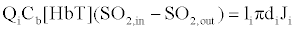(2)

where Qi is the mean BF in the ith segment. For a transient case, eq. (1) is further written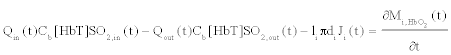(3)

in which Mi,HbO2 is the moles of oxygenated hemoglobin in the ith segment. According to the principle of mass balance, the third term on the left of Eq. (3) is actually the OCi of the ith segment (mole O2.s-1). Considering the fact that each molecular of hemoglobin is able to carry four molecular oxygen, Eq. (3) is stated,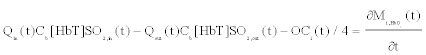(4)

Mass balance in tissues based on global analysis for estimating intravascular flux

The oxygen consumed by the tissues (organs) is supplied from three blood vessels sources: capillaries, arterioles and venules. As such, mass balance for O2 in the whole tissues (organ) yields for a steady case,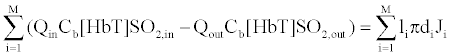(5)

and M is the number of blood vessels inside the tissues. Likewise, eq. (5) can be stated as if all the O2 is consumed,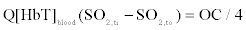(6)

For the dynamic case, eq. (6) is further written,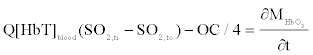(7)

where Q is the mean BF for all the blood vessels inside the tissues and is specified as the mean BF of tissues, [HbT]blood is the mean total blood hemoglobin concentration in the blood circulating through the tissues, OC is the mean oxygen consumption for the whole tissue volume Vtissue,MHbO2 is the molar amount of oxygenated hemoglobin inside the measurement volume, and SO2,ti , SO2,to is the averaged hemoglobin oxygen saturation at the inlet (artery) and outlet (vena) of the tissues, respectively. Moreover, it is noted that molar amount of oxygenated hemoglobin concentration of tissue is expressed as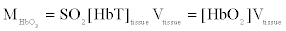(8)

Substituting Eq. (8) into (7), we obtain,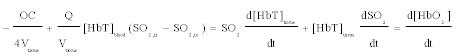(9)

where Vtissue is the tissue volume and is assumed constant here, and SO2 is the oxygen saturation of tissue. If the oxygen supply of tissues depends on the averaged oxygen saturation at the inlet and outlet of the tissues, tissue oxygen saturation should represent the weighted average of the arterial and venous saturation: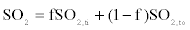(10)

Eq. (10) can be rewritten,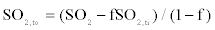(11)

Based on eq. (9) and (10), we get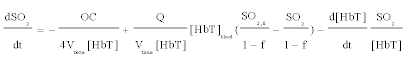(12)

Eq. (12) is the developed mathematical model that connects changes in BF and OC to known HbT and SO2 captured by DOT. As such, mean OC and BF can be recovered by fitting eq. (12) to time-resolved tissues oxygenation measurements. Eq. (12) is an ordinary partial differential equation that can be solved iteratively by Runge-Kutta 4th order method coupled with finite element method [26,27]. The fitting method is described as follows: with any given initial values for OC and BF within the specified range, this scheme is to optimize the OC and BF parameters based on the solution of eq. (12) to reach the minimized objective function in eq. (13).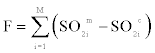(13)

in which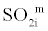is the measured oxygenation parameter from M discrete time points,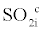is calculated oxygenation parameter from Eq. (12) for the same time points. Note the BF and OC are assumed constant during the measurements for the specified time range, due to the need for a sufficient time interval to obtain stable fitting results. Finally, it should point out that Carp et al. also used a very similar model as eq. (12) to analyze the dynamics response of compressed breast tissues though it seem that their model has no strong theoretical basis .

#### Results and Discussion

In this section, we show reconstruction results that demonstrate feasibility of the recovery of mean BF and OC based on the developed model described above. The fitting algorithm is evaluated using simulation test and small animal experiments.

Simulations are first conducted to validate the merits of the developed reconstruction algorithm for recovery BF and OC. For the test geometry, a circular background region (20 mm in radius) contains three circular targets (2 mm in radius each) positioned at 2 (top right), 9 (top left) and 6 o’clock (bottom), as displayed in figure 1a. The volume normalized BF (BF/Vtissue) and OC (OC/Vtissue) for the background media is 2.73×10-4 mL/mL/s and 3.283×10-3 μmol/mL/s, respectively, while the three inclusions have OC of 4.925×10-3 μmol/mL/s and BF of 6.825×10-4 mL/mL/s. The simulated oxygenation saturation signals were generated using eq. (12) with 2% random Gaussian noise added. The initial parameters are given by: HbTblood=0.72 mM, f=0.2, SO2,ti=0.98,27 HbTtarget=0.12 mM, HbTbackground=0.06 mM. The change of simulated oxygen saturation vs time is given in figures 1b and 1c for background media and the targets, respectively. The recovered BF and OC are shown in figures 1d and 1c, respectively.

Figure 1: Test geometry: (a) Generated simulated oxygenation measurement using eq. (12); (b) for the background; (c) targets; (d) Recovered BF image and (e) OC image.

It is observed in figure 1 that targets and background media are effectively recovered according to the position, size and quantitative BF and OC.

Animal experiments were performed using a dynamic DOT imaging system that has been described in detail elsewhere . To reconstruct BF and OC, the initial parameters are given by: HbTblood=0.72 mM, f=0.2, SO2,ti=0.98. The dynamic HbT and SO2 parameters are first calculated by fitted the reconstructed optical absorption coefficient using Beer’s law at wavelengths 633 nm, 760 and 853 . In addition, due to high nonlinear distribution of dynamic SO2, the SO2 distribution curve is then separated into several approximated linear segments to improve the fitting accuracy of BF and OC. Then mean BF and OC are fitted for each linear segment based on different initial values of HbT and SO2. In this investigation, there are 6 measurements at time 1, 2, 4, 6, 8 and 25 minutes. For each segment, only 2 two discrete oxygenation measurements are used for the fitting calculation. And we specify the fitted mean BF and OC for each segment as the values at the stating point of the segment. For example, we name the mean BF and OC fitted between time 1 and 2 minutes as the BF and OC at 1 minute.

Figures 2-6 present the reconstructed in vivo HbT, SO2, volume normalized BF and OC images. We see that the seizure is remarkably imaged with the highest contrast in HbT, SO2, volume normalized BF. In addition, the OC image is not effectively recovered due to insufficient time interval to obtain stable fitting results. Further, it is observed from the peak values of BF shown in figure 7 that the recovered blood flow values (3.9-36.9 ml/100 ml/min) are in good agreement with reported CBF of rats (between 10-120 ml/100ml/min) and human being (20-160 ml/100 ml/min) [30,31]. The in vivo results shown here further validate the merits of the developed mathematical model.

Figure 2: Reconstructed (a) SO2; (b) HbT (mM); (c) volume normalized BF (ml/ml/s) and (d) volume normalized OC (μmol/ml/s) images after 1 minute.

Figure 3: Reconstructed (a) SO2, (b) HbT (mM), (c) volume normalized BF (ml/ml/s), and (d) volume normalized OC (μmol/ml/s) images after 2 minutes.

Figure 4: Reconstructed (a) SO2, (b) HbT (mM), (c) volume normalized BF (ml/ml/s) and (d) volume normalized OC (μmol/ml/s) images after 4 minutes.

Figure 5: Reconstructed (a) SO2, (b) HbT (mM), (c) volume normalized BF (ml/ml/s) and (d) volume normalized OC (μmol/ml/s) images after 6 minutes.

Figure 6: Reconstructed (a) SO2, (b) HbT (mM), (c) volume normalized BF (ml/ml/s) and (d) volume normalized OC (μmol/ml/s) images after 8 minutes.

In summary, we have developed an effective model that is capable to estimate the OC and BF of biological tissues. However, this model can only provide mean vales of BF and OC during a time interval. And more measurements are required for this time interval to obtain stable and accurate fitting results. Our simulations and in vivo tests indicate at least 3 or 4 measurements for this time interval are needed to achieve reliable fitting computation of both BF and OC. In addition, highly nonlinear change of oxygen saturation during the time interval should be avoided to ensure the efficacy of the developed model. Ultimately, the assumption and utilization of constant tissues volume and other known tissues parameters will also affect the accuracy of the fitting calculation. In future, we plan to combine the laser speckle imaging setups with multiple wavelengths DOT imaging systems to quantitatively measure and image BF and OC of a local blood vessel network . Consequently, the developed imaging systems will enable us to accurately and quantitatively estimate the BF and OC of biological tissues based on the captured physiological parameters of tissues and vascular parameters from blood segments as well as the developed model.

#### References

Select your language of interest to view the total content in your interested language

### Article Usage

• Total views: 12136
• [From(publication date):
June-2013 - Aug 23, 2019]
• Breakdown by view type
• HTML page views : 8305
• PDF downloads : 3831

## Post your commentCan't read the image? click here to refresh
###### Peer Reviewed Journals

Make the best use of Scientific Research and information from our 700 + peer reviewed, Open Access Journals

International Conferences 2019-20

Meet Inspiring Speakers and Experts at our 3000+ Global Annual Meetings

Top